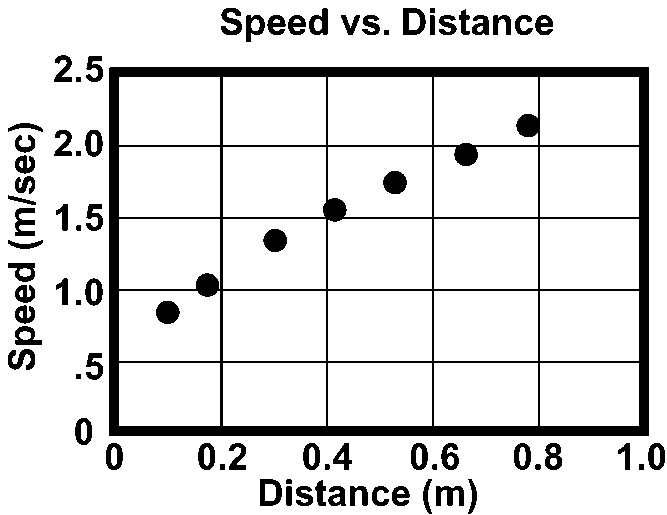# Physics: Chapter 3 Test

20 Questions | Attempts: 1070SettingsYou may not use any resources other than those provided by your teacher. If you go to any website other than this you will be given an immediate grade of 0, which will be very difficult to recover from and pass this class.

• 1.
A measure of how quickly an object gets from one place to another is:
• A.

Acceleration

• B.

Displacement

• C.

Speed

• D.

Position

• 2.
To elude a pack of wolves, an elk runs 15 kilometers in 0.75 hours. What is the speed of the elk?
• A.

5 km/hr

• B.

10 km/hr

• C.

12 km/hr

• D.

20 km/hr

• 3.
Brett Favre throws a football to his receiver 42 yards downfield. If it takes 1.2 seconds to reach the receiver, how fast is the football traveling in yards per second?
• A.

50

• B.

35

• C.

29

• D.

0.29

• 4.
A golfer walks at an average rate of 7.20 feet per second on the golf course. How much time is required for her to walk from the tee to the green if the distance is 612 feet?
• A.

0.0118 minutes

• B.

4406 seconds

• C.

0.85 minutes

• D.

85 seconds

• 5.
The expression “per” as in miles per hour or meters per second mathematically indicates:
• A.

• B.

Subtraction

• C.

Multiplication

• D.

Division

• 6.
Distance may be expressed as a:
• A.

Ratio of time to speed.

• B.

Ratio of speed to time.

• C.

Product of time and speed.

• D.

quotient of time and speed.

• 7.
Speed may be expressed as a:
• A.

Ratio of distance to time.

• B.

Ratio of time to distance.

• C.

Product of distance and time.

• D.

Product of time and distance.

• 8.
How far will a Canada goose travel if it flies at an average speed of 66 miles per hour for 20 minutes?
• A.

132 miles

• B.

22 miles

• C.

13.2 miles

• D.

3.3 miles

• 9.
On this graph, the dependent variable is:
• A.

Distance

• B.

Speed

• C.

Acceleration

• D.

The slope of the speed vs. distance graph

• 10.
The term that refers to the location of an object is:
• A.

Distance

• B.

Displacement

• C.

Position

• D.

All of the above

• 11.
The term that describes the amount of change in a certain direction in the location of an object is:
• A.

Distance

• B.

Displacement

• C.

Position

• D.

None of the above

• 12.
The total length of a journey from start to finish with no regard to direction is called:
• A.

Distance

• B.

Displacement

• C.

Position

• D.

Velocity

• 13.
The slope of a distance versus time graph represents:
• A.

Position

• B.

Displacement

• C.

Speed

• D.

Acceleration

• 14.
Each of the four graphs pictured in the diagram below represents the motion of four different objects.   Figure 3-1 Which graph in Figure 3-1 represents an object whose speed is zero?
• A.

1

• B.

2

• C.

3

• D.

4

• 15.
Each of the four graphs pictured in the diagram below represents the motion of four different objects.   Figure 3-1 Which graph in Figure 3-1 represents an object with non-zero uniform speed?
• A.

1

• B.

2

• C.

3

• D.

4

• 16.
Each of the four graphs pictured in the diagram below represents the motion of four different objects.   Figure 3-1 Which graph in Figure 3-1 represents an object whose speed is increasing?
• A.

1

• B.

2

• C.

3

• D.

4

• 17.
Each of the four graphs pictured in the diagram below represents the motion of four different objects.   Figure 3-1 Which graph in Figure 3-1 represents an object whose speed is decreasing to zero?
• A.

1

• B.

2

• C.

3

• D.

4

• 18.
The slope of a graph line is determined by calculating the:
• A.

Change in the horizontal values divided by the change in the vertical values.

• B.

Change in the horizontal values multiplied by the change in the vertical values.

• C.

Change in the vertical values divided by the change in the horizontal values.

• D.

Change in the vertical values multiplied by the change in the horizontal values.

• 19.
The graph below represents the motion of a cart along a straight line. During which time intervals is the cart moving with non-zero uniform speed?
• A.

AB and BC

• B.

AB and CD

• C.

BC and CD

• D.

BC and DE

• 20.
Each of the four displacement vs. time graphs pictured in the diagram below represents the motion of four different objects. Figure 3-2 A ball is thrown vertically upward from the surface of the Earth. The ball returns to Earth. Which graph in Figure 3-2 represents the displacement of the ball according to this description?
• A.

1

• B.

2

• C.

3

• D.

4

## Related TopicsBack to top
×

Wait!
Here's an interesting quiz for you.